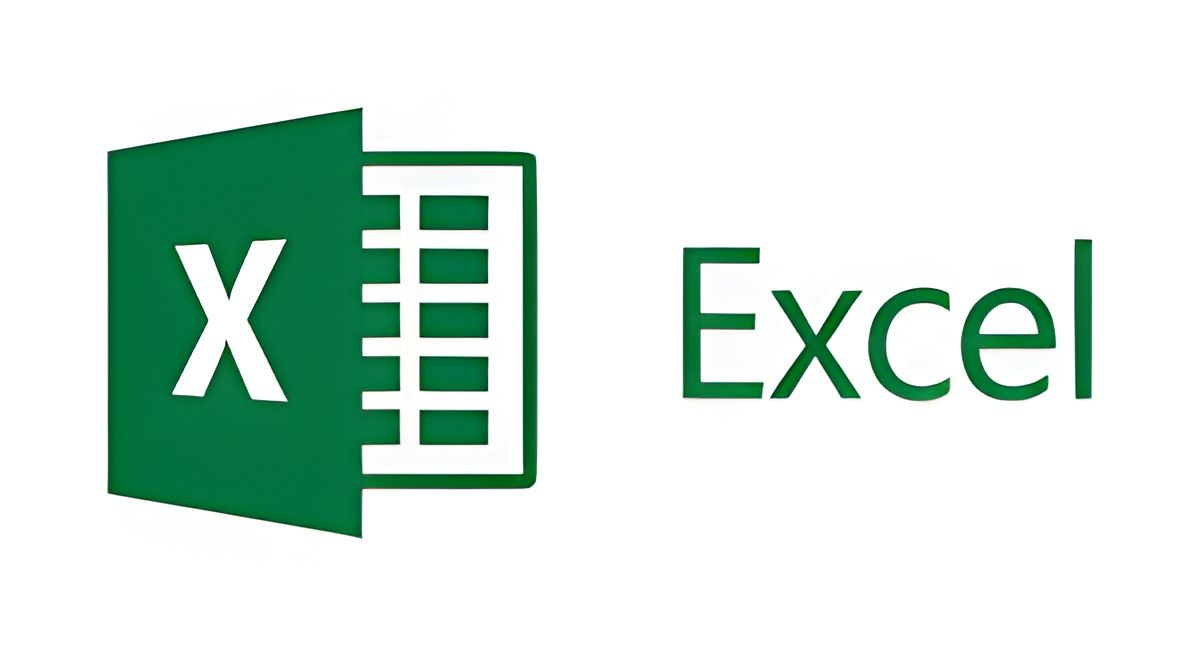# Boost Your Excel Skills: 26 Functions Every User Should Know

## From basic calculations to advanced data analysis, these 26 Excel functions are must-knows for anyone aiming to become more productive and efficient in Excel.Monday May 22, 2023,

With an impressive 1 billion users and a penetration rate of 99% within the world's businesses, Microsoft Excel has become an essential tool in almost every office and industry. Excel's functions enable users to manipulate, calculate and analyse data with incredible efficiency. Whether you're a beginner or a seasoned Excel user, understanding these 26 must-know Excel functions will significantly enhance your ability to manage and interpret data.

## Looking Up and Retrieving Data

1. =VLOOKUP: This function allows you to search for a value in a table and return a corresponding value from the same row.
2. =INDEX/MATCH: These functions allow users to perform advanced lookups and retrieve data from a table or range of cells.
3. =INDEX: This function enables you to return the value of a cell in a specified row and column.
4. =MATCH: This function searches for a specified value in a range of cells and returns the position of the value within that range.

## Logical and Conditional Functions

1. =IF: This function lets you specify a logical test and then take different actions based on whether the test is true or false.
2. =SUMIF: This function sums the values in a range that meet a certain criteria.
3. =SUMIFS: This function sums the values in a range that meet multiple criteria.
4. =COUNTIF: This function allows users to count the number of cells that meet specified criteria.
5. =COUNTIFS: This function counts the number of times a criteria is met.

## Basic Calculation Functions

1. =SUM: This function allows you to quickly add up a range of cells.
2. =COUNT: This function counts the number of cells in a range that contain numbers.
3. =AVERAGE: This function calculates the average of a range of cells.
4. =MAX: This function returns the largest value in a range of cells.
5. =MIN: This function returns the smallest value in a range of cells.

## Data Transformation Functions

1. =CONVERT: This function converts one unit of measurement to another, like meters to feet or Celsius to Fahrenheit.
2. =CONCATENATE: This function allows you to combine multiple text strings into one string.
3. =TRIM: This function helps to remove the extra spaces in data. It is particularly useful in cleaning up irregular spacing from imported data.

## Visual Representation and Analysis

1. SPARKLINES: This function allows you to insert mini-charts inside any cell, providing a visual representation of data trends or patterns.
2. GOAL SEEK: Also known as What-if-Analysis, Goal Seek uses trial and error to back-solve a problem by plugging in guesses until it arrives at the correct answer.
3. PivotTable: This function enables you to analyse vast amounts of data effortlessly, summarising it into a format that's easier to understand.

1. =CHOOSE: This function allows you to select a value by its index number, providing a simple way to make conditional choices.
2. =SUBTOTAL: This function calculates subtotals for a range of cells or a list, useful in financial and statistical operations.
3. =NETWORKDAYS: This function calculates the number of working days between two dates, taking weekends and optionally specified holidays into account.
4. =OFFSET: This function creates dynamic reference ranges, which can adjust automatically when new data is added.
5. =HYPERLINK: This function creates clickable links within your Excel document, connecting to webpages, files, or other parts of